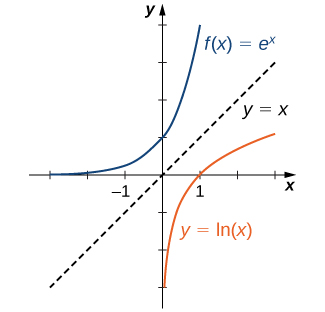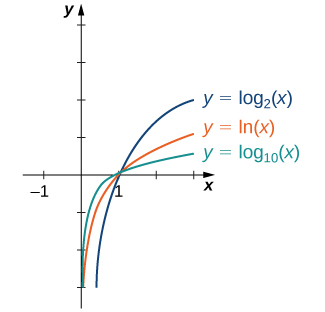# 1.5 Exponential and logarithmic functions  (Page 4/17)

 Page 4 / 17

The exponential function $f\left(x\right)={b}^{x}$ is one-to-one, with domain $\left(\text{−}\infty ,\infty \right)$ and range $\left(0,\infty \right).$ Therefore, it has an inverse function, called the logarithmic function with base $b.$ For any $b>0,b\ne 1,$ the logarithmic function with base b , denoted ${\text{log}}_{b},$ has domain $\left(0,\infty \right)$ and range $\left(\text{−}\infty ,\infty \right),$ and satisfies

${\text{log}}_{b}\left(x\right)=y\phantom{\rule{0.2em}{0ex}}\text{if and only if}\phantom{\rule{0.2em}{0ex}}{b}^{y}=x.$

For example,

$\begin{array}{cccc}{\text{log}}_{2}\left(8\right)=3\hfill & & & \text{since}\phantom{\rule{3em}{0ex}}{2}^{3}=8,\hfill \\ {\text{log}}_{10}\left(\frac{1}{100}\right)=-2\hfill & & & \text{since}\phantom{\rule{3em}{0ex}}{10}^{-2}=\frac{1}{{10}^{2}}=\frac{1}{100},\hfill \\ {\text{log}}_{b}\left(1\right)=0\hfill & & & \text{since}\phantom{\rule{3em}{0ex}}{b}^{0}=1\phantom{\rule{0.2em}{0ex}}\text{for any base}\phantom{\rule{0.2em}{0ex}}b>0.\hfill \end{array}$

Furthermore, since $y={\text{log}}_{b}\left(x\right)$ and $y={b}^{x}$ are inverse functions,

${\text{log}}_{b}\left({b}^{x}\right)=x\phantom{\rule{0.2em}{0ex}}\text{and}\phantom{\rule{0.2em}{0ex}}{b}^{{\text{log}}_{b}\left(x\right)}=x.$

The most commonly used logarithmic function is the function ${\text{log}}_{e}.$ Since this function uses natural $e$ as its base, it is called the natural logarithm    . Here we use the notation $\text{ln}\left(x\right)$ or $\text{ln}\phantom{\rule{0.1em}{0ex}}x$ to mean ${\text{log}}_{e}\left(x\right).$ For example,

$\text{ln}\left(e\right)={\text{log}}_{e}\left(e\right)=1,\text{ln}\left({e}^{3}\right)={\text{log}}_{e}\left({e}^{3}\right)=3,\text{ln}\left(1\right)={\text{log}}_{e}\left(1\right)=0.$

Since the functions $f\left(x\right)={e}^{x}$ and $g\left(x\right)=\text{ln}\left(x\right)$ are inverses of each other,

$\text{ln}\left({e}^{x}\right)=x\phantom{\rule{0.2em}{0ex}}\text{and}\phantom{\rule{0.2em}{0ex}}{e}^{\text{ln}\phantom{\rule{0.1em}{0ex}}x}=x,$

and their graphs are symmetric about the line $y=x$ ( [link] ).The functions y = e x and y = ln ( x ) are inverses of each other, so their graphs are symmetric about the line y = x .

At this site you can see an example of a base-10 logarithmic scale.

In general, for any base $b>0,b\ne 1,$ the function $g\left(x\right)={\text{log}}_{b}\left(x\right)$ is symmetric about the line $y=x$ with the function $f\left(x\right)={b}^{x}.$ Using this fact and the graphs of the exponential functions, we graph functions ${\text{log}}_{b}$ for several values of $b>1$ ( [link] ).Graphs of y = log b ( x ) are depicted for b = 2 , e , 10 .

Before solving some equations involving exponential and logarithmic functions, let’s review the basic properties of logarithms.

## Rule: properties of logarithms

If $a,b,c>0,b\ne 1,$ and $r$ is any real number, then

$\begin{array}{cccc}1.\phantom{\rule{2em}{0ex}}{\text{log}}_{b}\left(ac\right)={\text{log}}_{b}\left(a\right)+{\text{log}}_{b}\left(c\right)\hfill & & & \text{(Product property)}\hfill \\ 2.\phantom{\rule{2em}{0ex}}{\text{log}}_{b}\left(\frac{a}{c}\right)={\text{log}}_{b}\left(a\right)-{\text{log}}_{b}\left(c\right)\hfill & & & \text{(Quotient property)}\hfill \\ 3.\phantom{\rule{2em}{0ex}}{\text{log}}_{b}\left({a}^{r}\right)=r{\text{log}}_{b}\left(a\right)\hfill & & & \text{(Power property)}\hfill \end{array}$

## Solving equations involving exponential functions

Solve each of the following equations for $x.$

1. ${5}^{x}=2$
2. ${e}^{x}+6{e}^{\text{−}x}=5$
1. Applying the natural logarithm function to both sides of the equation, we have
$\text{ln}{5}^{x}=\text{ln}\phantom{\rule{0.1em}{0ex}}2.$

Using the power property of logarithms,
$x\phantom{\rule{0.1em}{0ex}}\text{ln}\phantom{\rule{0.1em}{0ex}}5=\text{ln}\phantom{\rule{0.1em}{0ex}}2.$

Therefore, $x=\text{ln}\phantom{\rule{0.1em}{0ex}}2\text{/}\text{ln}\phantom{\rule{0.1em}{0ex}}5.$
2. Multiplying both sides of the equation by ${e}^{x},$ we arrive at the equation
${e}^{2x}+6=5{e}^{x}.$

Rewriting this equation as
${e}^{2x}-5{e}^{x}+6=0,$

we can then rewrite it as a quadratic equation in ${e}^{x}\text{:}$
${\left({e}^{x}\right)}^{2}-5\left({e}^{x}\right)+6=0.$

Now we can solve the quadratic equation. Factoring this equation, we obtain
$\left({e}^{x}-3\right)\left({e}^{x}-2\right)=0.$

Therefore, the solutions satisfy ${e}^{x}=3$ and ${e}^{x}=2.$ Taking the natural logarithm of both sides gives us the solutions $x=\text{ln}\phantom{\rule{0.1em}{0ex}}3,\text{ln}\phantom{\rule{0.1em}{0ex}}2.$

Solve ${e}^{2x}\text{/}\left(3+{e}^{2x}\right)=1\text{/}2.$

$x=\frac{\text{ln}\phantom{\rule{0.1em}{0ex}}3}{2}$

## Solving equations involving logarithmic functions

Solve each of the following equations for $x.$

1. $\text{ln}\left(\frac{1}{x}\right)=4$
2. ${\text{log}}_{10}\sqrt{x}+{\text{log}}_{10}x=2$
3. $\text{ln}\left(2x\right)-3\phantom{\rule{0.1em}{0ex}}\text{ln}\left({x}^{2}\right)=0$
1. By the definition of the natural logarithm function,
$\text{ln}\left(\frac{1}{x}\right)=4\phantom{\rule{0.2em}{0ex}}\text{if and only if}\phantom{\rule{0.2em}{0ex}}{e}^{4}=\frac{1}{x}.$

Therefore, the solution is $x=1\text{/}{e}^{4}.$
2. Using the product and power properties of logarithmic functions, rewrite the left-hand side of the equation as
${\text{log}}_{10}\sqrt{x}+{\text{log}}_{10}x={\text{log}}_{10}x\sqrt{x}={\text{log}}_{10}{x}^{3\text{/}2}=\frac{3}{2}{\text{log}}_{10}x.$

Therefore, the equation can be rewritten as
$\frac{3}{2}{\text{log}}_{10}x=2\phantom{\rule{0.2em}{0ex}}\text{or}\phantom{\rule{0.2em}{0ex}}{\text{log}}_{10}x=\frac{4}{3}.$

The solution is $x={10}^{4\text{/}3}=10\sqrt{10}.$
3. Using the power property of logarithmic functions, we can rewrite the equation as $\text{ln}\left(2x\right)-\text{ln}\left({x}^{6}\right)=0.$
Using the quotient property, this becomes
$\text{ln}\left(\frac{2}{{x}^{5}}\right)=0.$

Therefore, $2\text{/}{x}^{5}=1,$ which implies $x=\sqrt{2}.$ We should then check for any extraneous solutions.

any genius online ? I need help!!
Pina
need to learn polynomial
Zakariya
i will teach...
nandu
I'm waiting
Zakariya
evaluate the following computation (x³-8/x-2)
teach me how to solve the first law of calculus.
what is differentiation
f(x) = x-2 g(x) = 3x + 5 fog(x)? f(x)/g(x)
fog(x)= f(g(x)) = x-2 = 3x+5-2 = 3x+3 f(x)/g(x)= x-2/3x+5
diron
pweding paturo nsa calculus?
jimmy
how to use fundamental theorem to solve exponential
find the bounded area of the parabola y^2=4x and y=16x
what is absolute value means?
Chicken nuggets
Hugh
🐔
MM
🐔🦃 nuggets
MM
(mathematics) For a complex number a+bi, the principal square root of the sum of the squares of its real and imaginary parts, √a2+b2 . Denoted by | |. The absolute value |x| of a real number x is √x2 , which is equal to x if x is non-negative, and −x if x is negative.
Ismael
find integration of loge x
find the volume of a solid about the y-axis, x=0, x=1, y=0, y=7+x^3
how does this work
Can calculus give the answers as same as other methods give in basic classes while solving the numericals?
log tan (x/4+x/2)
Rohan
Rohan
y=(x^2 + 3x).(eipix)
Claudia
Ismael
A Function F(X)=Sinx+cosx is odd or even?
neither
David
Neither
Lovuyiso
f(x)=1/1+x^2 |=[-3,1]
apa itu?
fauzi
determine the area of the region enclosed by x²+y=1,2x-y+4=0
Hi
MP
Hi too
Vic
hello please anyone with calculus PDF should share
Which kind of pdf do you want bro?
Aftab
hi
Abdul
can I get calculus in pdf
Abdul
explain for me
Usman
okay I have such documents
Fitzgerald
Hamza

#### Get Jobilize Job Search Mobile App in your pocket Now!By OpenStaxBy Marriyam RanaBy Brooke DelaneyBy Stephen VoronBy OpenStaxBy Richley CrapoBy Ryan LoweBy Brooke DelaneyByBy OpenStax